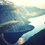# I can't find any mistake here

Let, $$S_\infty=1-\frac{1}{2}+\frac{1}{3}-\frac{1}{4}+\frac{1}{5}+....$$

By calculating, we find that:

$S_1=1$

$S_2=\frac{1}{2}$

$S_3=0.833333...$

$S_4=0.5833333...$

$S_5=0.78333...$

$S_6=0.616666...$

$S_7=0.759523809...$

$S_8=0.634523809...$

$S_9=0.7445492...$

$S_{10}=0.64563492...$

We'll notice that the $S_n\rightarrow0.7$ as $n\rightarrow\infty$ and in fact, it is $\ln 2=0.69314718...$

Now, $S_\infty=1-\frac{1}{2}+\frac{1}{3}-\frac{1}{4}+\frac{1}{5}+....$

And $2S_\infty=2-1+\frac{2}{3}-\frac{2}{4}+\frac{2}{5}-\frac{2}{6}+\frac{2}{7}-\frac{2}{8}+\frac{2}{9}-\frac{2}{10}+...$

$=1+\frac{2}{3}-\frac{1}{2}+\frac{2}{5}-\frac{1}{3}+\frac{2}{7}-\frac{1}{4}+\frac{2}{9}-\frac{1}{5}+...$

$=1-\frac{1}{2}+(\frac{2}{3}-\frac{1}{3})-\frac{1}{4}+(\frac{2}{5}-\frac{1}{5})-...$

$=1-\frac{1}{2}+\frac{1}{3}-\frac{1}{4}+\frac{1}{5}+...$

$=S_\infty$

So, $2S_\infty=S_\infty$

We know that, $S_\infty$ is not equal to $0$.

Then $2=1$!!Note by Fahim Muhtamim
1 year, 5 months ago

This discussion board is a place to discuss our Daily Challenges and the math and science related to those challenges. Explanations are more than just a solution — they should explain the steps and thinking strategies that you used to obtain the solution. Comments should further the discussion of math and science.

When posting on Brilliant:

• Use the emojis to react to an explanation, whether you're congratulating a job well done , or just really confused .
• Ask specific questions about the challenge or the steps in somebody's explanation. Well-posed questions can add a lot to the discussion, but posting "I don't understand!" doesn't help anyone.
• Try to contribute something new to the discussion, whether it is an extension, generalization or other idea related to the challenge.

MarkdownAppears as
*italics* or _italics_ italics
**bold** or __bold__ bold
- bulleted- list
• bulleted
• list
1. numbered2. list
1. numbered
2. list
Note: you must add a full line of space before and after lists for them to show up correctly
paragraph 1paragraph 2

paragraph 1

paragraph 2

[example link](https://brilliant.org)example link
> This is a quote
This is a quote
    # I indented these lines
# 4 spaces, and now they show
# up as a code block.

print "hello world"
# I indented these lines
# 4 spaces, and now they show
# up as a code block.

print "hello world"
MathAppears as
Remember to wrap math in $$ ... $$ or $ ... $ to ensure proper formatting.
2 \times 3 $2 \times 3$
2^{34} $2^{34}$
a_{i-1} $a_{i-1}$
\frac{2}{3} $\frac{2}{3}$
\sqrt{2} $\sqrt{2}$
\sum_{i=1}^3 $\sum_{i=1}^3$
\sin \theta $\sin \theta$
\boxed{123} $\boxed{123}$

Sort by:

Rearranging the terms of a sum that is not absolutely convergent may change the value of the sum. In fact, there is a wonderful result that if a series is convergent but not absolutely convergent, then for any real number $r,$ you can rearrange the terms of the series so that it sums to $r.$ Here is a nice reference.

- 1 year, 5 months ago

Your mistake is when you put it as $1-\frac{1}{2}+(\frac{2}{3}-\frac{1}{3})..$.

The gaps between $\frac{2}{3} and \frac{1}{3}, \frac{2}{5}and \frac{1}{5},\frac{2}{9} and \frac{1}{9}$. Increase each time. With this logic you can prove that there are twice as many even numbers as odd numbers.

$1,3,2,5,7,4,9,11,6..$

- 1 year, 5 months ago